# How to Compare Two Cells in ExcelAside from staring at them closely, how can you compare two cells in Excel? Here are a few functions and formulas that check the contents of two cells, to see if they are the same. We'll start with a simple check, then move up the formula ladder, for more complex comparisons.

### Easy Way to Compare Two Cells

The quickest way to compare two cells is with a formula that uses the equal sign.

• =A2=B2

If the cell contents are the same, the result is TRUE. (Upper and lower case versions of the same letter are treated as equal).### Ignore Extra Spaces

If you just want to compare two cells, but aren't concerned about leading spaces, trailing spaces, or extra spaces, use the TRIM function to remove them, for one or both of the cells.

• =TRIM(A2)=TRIM(B2)

That can help if you're trying to match text strings to the values in an imported list, such as this VLOOKUP example.

### Compare Two Cells Exactly

If you need to compare two cells for contents and upper/lower case, use the EXACT function. As its name indicate, that function can check for an exact match between text strings, including upper and lower case. It doesn't test the formatting though, so it won't detect if one cell has some or all of the characters in bold, and the other cell doesn't.

• =EXACT(A2,B2)

See more EXACT function examples in my 30 Excel Functions series.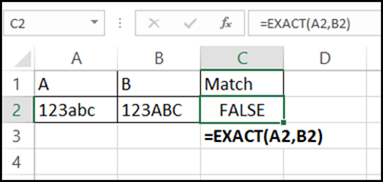### Partially Compare Two Cells

Sometimes you don't need a full comparison of two cells – you just need to check the first few characters, or a 3-digit code at the end of a string.

To compare characters at the beginning of the cells, use the LEFT function. For example, check the first 3 characters:

• =LEFT(A2,3)=LEFT(B2,3)

To compare characters at the end of the cells, use the RIGHT function. For example, check the last 3 characters:

• =RIGHT(A2,3)=RIGHT(B2,3)

You can combine LEFT or RIGHT with TRIM, if you're not concerned about the space characters:

• =RIGHT(TRIM(A2),3)=RIGHT(TRIM(B2),3)

And combine LEFT or RIGHT with EXACT, to check if upper/lower case match too. This formula will ignore extra spaces, but checks the case:

• =EXACT(RIGHT(TRIM(A2),3),RIGHT(TRIM(B2),3))

### How Much Do Cells Match?

Finally, here's a formula from UniMord, who needs to know how much of a match there is between two cells. Are the first 5 characters the same? The first 10? What percent of the string in A2, starting from the left, is matched in cell B2?

Here's a sample list, where the addresses in column A and B and being compared.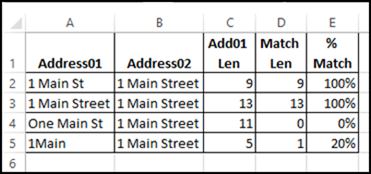### Get the Text Length

The first step in calculating the percent that the cells match is to find the length of the address in column A. This formula is in cell C2:

• =LEN(A2)

### Get the Match Length

The formula in column D is doing the hard work. It finds how many characters, starting from the left in each cell, are a match. Lower and upper case are not compared.

• =SUMPRODUCT(
--(LEFT(A3,
ROW(INDIRECT("A1:A" & C3)))
=LEFT(B3,
ROW(INDIRECT("A1:A" &C3)))))

### How the Match Len Formula Works

The INDIRECT function creates a reference to a range of cells, starting from cell A1. The range ends in column A, in the row that matches the length calculated in column C. So, in row 2, that range is A1:A9.

The ROW function returns the row for each of the rows in that range. That's why we use ROW/INDIRECT, instead of just referring to the length in cell C2.

In this screen shot, I've used the F9 key to calculate that part of the formula, and you can see the row numbers.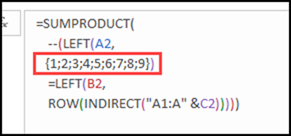Then, the LEFT functions return the characters that are 1, 2, 3…characters to the left in each cell. In this screen shot, I've calculated both of the LEFT functions, and you can see that there is a match for lengths 1 through 9.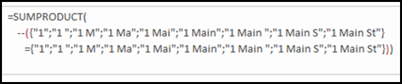However, if I do the same thing in row 5, only the first character is a match. After that, the characters are different in the two cells.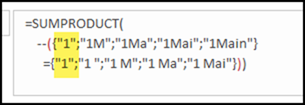The equal sign compares the values for characters 1 through 5 in this example, and returns TRUE if they match, and FALSE if they do not match.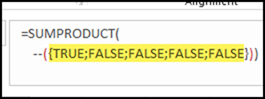The double minus sign converts each TRUE to a 1, and each FALSE to a zero.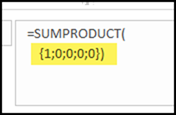Finally, the SUMPRODUCT function adds up those numbers, to give the number of characters, from the left, that match. In row 5, that total is 1### Get the Percent Match

Once the length and match length have been calculated, it's easy to find the percent matched. This formula is in cell E2, to compare the lengths:

• =D2/C2

There is a 100% match in row 2, and only a 20% match, starting from the left, in row 5.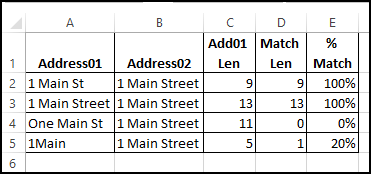Thanks, UniMord, for sharing your formula to compare two cells, character by character.

### More Ways to Compare Two Cells

Here are a few more articles that show examples of how to compare two cells – either the full content, or partial content.

_____________________________________________

## 10 thoughts on “How to Compare Two Cells in Excel”

1. I'm trying to compare 2 columns with Yes or No comments in them, using the IF function, but want to differentiate between the columns where the comments are Yes/Yes, Yes/No (either way) and No/No (with the results - same (True) or different (False) going into a third column. So far I've managed to work out how to get those that are different (Yes/No) or the same (Yes/Yes and No/No) but need to be able to separate the Yes/Yes and No/No as while they have matched responses, one is good and one is bad!

An ideas gratefully received!
PQ

1. Pauline, if you count the Yes comments, that might do what you need. For example, if the comments are in B2 and C2:
=COUNTIF(B2:C2,"Yes")

1. Jaime says:

Hi Debra -

How can I compare more than two cells? I used this formula but is not accurate. I don't really need it to be case sensitive so I also remove 'EXACT', still didn't work.
=AND(EXACT(B23,K23),EXACT(B23,M23),EXACT(B23,Q23),EXACT(B23,V23))

I managed to compare two cells using this formula but adding more cells gave me an error.
=IF(OR(B15=Q15, OR(B15=V15)), "Y", "N")

Any recommendation is greatly appreciated!

2. Jaime, your formula worked when I tried it. If you're getting an unexpected result, perhaps some cells have space characters, or other hidden items.
If you don't need case sensitivity, you could also use this:
=AND(B23=K23,B23=M23,B23=Q23,B23=V23)

2. Pauline Quennell says:

Many thanks, Debra
Best wishes
PQ

3. Ansari says:

Hi Debra,

I am looking to find the total number of mismatched between two texts. Your solution above will stop at the first mismatch and then consider all characters after that as mismatch. But is there a way to compare letter by letter.
In cell A1 I have Richard and cell B1 I have Rickard . So in Cell C1 i would like to 1 since only character mismatch
A1 - 123abcd456 B1 = 14xy456 C1 = 9 . Since 9 character from A1 is not matching with B1. IS there a way to do this. Please help

4. Jaime says:

Hello -
If I need to compare a total of 5 cells and not case sensitive. What is the best way? I tried this but it doesn't work, I also took out 'EXACT'
=AND(EXACT(B23,K23),EXACT(B23,M23),EXACT(B23,Q23),EXACT(B23,V23))

I was able to compare with 2 cells with this formula but adding the others gave me an error message.

=IF(OR(B17=Q17, OR(B17=V17)), "Y", "N")

5. Jaime says:

Thank you Debra!!
Another question 🙂
How would I write the formula if at least one of the cell is a match to B23, then return True? I don't need to have all the compare cells to match in order it to be True, one match will do.

6. Jaime says:

Hi Debra, please disregard my previous post as I used the formula below, it work on majority of my sheet except three cells, which return as True but it's not accurate because B23 is Blank. I checked the format of the cells and it appear to be consistent with the others so I don't know why these three cells result is like this. It should be False as the result.

=OR(B23=K23,B23=M23,B23=Q23,B23=V23)

7. Roy says:

Is there a way to do this?

I am looking to find the total number of mismatched between two texts. Your solution above will stop at the first mismatch and then consider all characters after that as mismatch. But is there a way to compare letter by letter.
In cell A1 I have Richard and cell B1 I have Rickard . So in Cell C1 i would like to 1 since only character mismatch
A1 - 123abcd456 B1 = 14xy456 C1 = 9 . Since 9 character from A1 is not matching with B1. IS there a way to do this. Please help

This site uses Akismet to reduce spam. Learn how your comment data is processed.